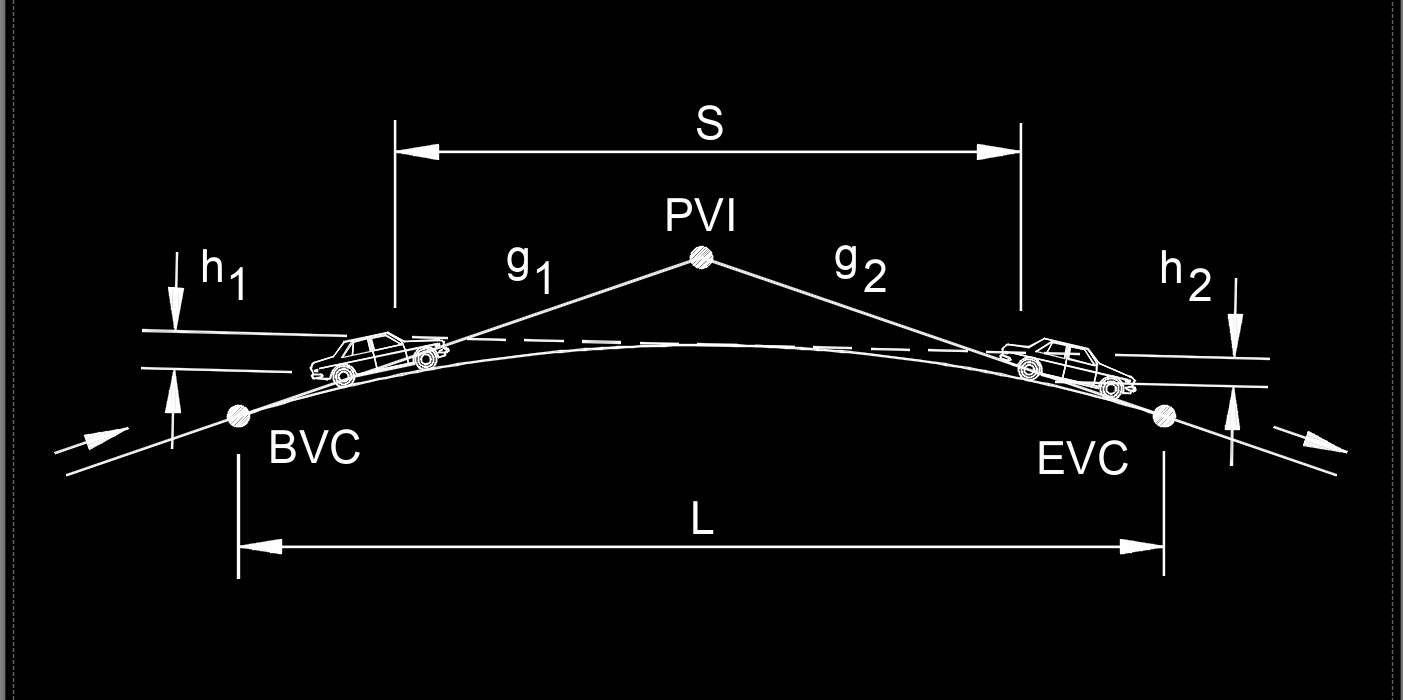# Length of Crest Vertical Curve for Stopping Sight Distance

Written by Jerry Ratzlaff on . Posted in Civil EngineeringLength of vertical curve (crest vertical curve), abbreviated as L, is the sight distance related to the curve length.  Crest and sag vertical curves are in the shape of a parabola.

## Length of Crest vertical curve for stopping Sight Distance formulas

 $$\large{ L = \frac{ A \; S^2 }{ 100 \; \left( \sqrt{ 2\;h_1 } \;+\; \sqrt{ 2\;h_2 } \right)^2 } }$$ ($$S \;<\; L$$) (crest vertical curve) $$\large{ L = 2 \; S \;-\; \frac{ 200 \; \left( \sqrt{ h_1 } \;+\; \sqrt{ h_2 } \right)^2 }{ A } }$$ ($$S \;>\; L$$) (crest vertical curve) $$\large{ L = \frac{ A \; S^2 }{ 2158 } }$$ ($$S \;<\; L$$) (when the height of eye and the height of object are 3.5' and 2' respectively, as uesd for breaking distance) (crest vertical curve) $$\large{ L = 2 \; S \;-\; \frac{ 2158 }{ A } }$$ ($$S \;>\; L$$) (when the height of eye and the height of object are 3.5' and 2' respectively, as uesd for breaking distance) (crest vertical curve) $$\large{ L = K \; A }$$ (crest vertical curve)

### Where:

$$\large{ L }$$ = length of vertical curve

$$\large{ A }$$ = algebric difference in grade (%)

$$\large{ h_1 }$$ = height of drivers' eyes above the roadway surface

$$\large{ h_2 }$$ = height of object above the roadway surface

$$\large{ K }$$ = K value

$$\large{ S }$$ = sight distance for stopping or passing